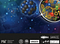Going to smaller and smaller distance scales reveals more fundamental views of nature, which means if we can understand and describe the smallest scales, we can build our way to an understanding of the largest ones. We do not know whether there is a lower limit to how small ‘chunks of space’ can be. (PERIMETER INSTITUTE)

# This Is Why Space Needs To Be Continuous, Not Discrete

## We might live in a quantum Universe, but we’ll violate the principle of relativity if space is discrete.

If you try and divide matter into smaller and smaller chunks, you’ll eventually arrive at the particles we know of as fundamental: the ones that cannot be decomposed any further. The particles of the Standard Model — quarks, charged leptons, neutrinos, bosons, and their antiparticle counterparts — are the indivisible entities that account for every directly measured particle in our Universe. They are not only fundamentally quantum, but discrete.

If you take any system made up of matter, you could literally count the number of quantum particles in your system and always wind up with the same answer. But that’s not true, as far as we can tell, of the space that those particles occupy. Observationally and experimentally, there’s no evidence for a “smallest” length scale in the Universe, but there’s an even bigger theoretical objection. If space is discrete, then the principle of relativity is wrong. Here’s why.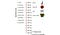The objects we’ve interacted with in the Universe range from very large, cosmic scales down to about 10^-19 meters, with the newest record set by the LHC. There’s a long, long way down (in size) and up (in energy) to either the scales that the hot Big Bang achieves, or the Planck scale, which is around 10^-35 meters. (UNIVERSITY OF NEW SOUTH WALES / SCHOOL OF PHYSICS)

Just as you can learn what matter is made of by dividing it up into smaller chunks until you get something indivisible, you might intuit that you could do the same thing to space. Perhaps there’s some smallest scale you could eventually reach where you could divide it no further: some smallest unit of space on the smallest scales.

If this were the case, our notions of a continuous Universe would only be an illusion. Particles instead would jump from one discrete location to the next, perhaps in discrete chunks of time as well. The speed of light would be the cosmic speed limit at which those jumps occur: you can move no faster than one unit of space in a given period of time. Instead of motion through space and time flowing freely from one location and moment to the next, they’d only appear to do so on the large, many-jump scales we’re capable of perceiving.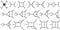Today, Feynman diagrams are used in calculating every fundamental interaction spanning the strong, weak, and electromagnetic forces, including in high-energy and low-temperature/condensed conditions. The particles and fields are both quantized in quantum field theory, and beta decay proceeds just fine without a minimum length scale. Perhaps a quantum theory of gravity will remove the need for a minimum length scale in all quantum calculations. (DE CARVALHO, VANUILDO S. ET AL. NUCL.PHYS. B875 (2013) 738–756)

Today, we have two separate theories that govern how the Universe works: quantum physics, which governs the electromagnetic and nuclear forces, and General Relativity, which governs the gravitational force. Although we fully expect that there ought to be a quantum theory of gravity — there must be if we ever hope to answer questions like “what happens to the gravitational field of an electron as it goes through a double slit?” — we don’t know what it looks like.

But one possibility that’s often floated is that a quantum theory of gravity could lead to a discrete structure for space and time, which approaches like Loop Quantum Gravity require. But the notion of space and/or time being broken up into finite, indivisible chunks didn’t start there. It’s an idea that first arose nearly a century ago, with Heisenberg finding its origins in the idea of the quantum Universe itself.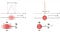An illustration between the inherent uncertainty between position and momentum at the quantum level. There is a limit to how well you can measure these two quantities simultaneously, as multiplying those two uncertainties together can yield a value that must be larger than a certain finite amount. When one is known more accurately, the other is inherently less able to be known with any degree of meaningful accuracy. (E. SIEGEL / WIKIMEDIA COMMONS USER MASCHEN)

Heisenberg is most famous for the uncertainty principle, which is a fundamental limitation on how precisely you can measure-and-know two different properties of a system simultaneously. For example, these fundamental limits apply to:

• position and momentum,

But Heisenberg also demonstrated that when we tried to promote our quantum theories of individual particles to fully quantum field theories, some of the probability calculations we’d perform would give nonsense answers, like “infinite” or “negative” probabilities for certain outcomes. (Remember, all probabilities must always be between 0 and 1.)

It was here that his brilliant stroke came in: if you postulated that space wasn’t continuous, but instead had some minimum distance scale inherent to it, those infinities disappeared.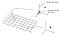If you confine a particle to a space, and try to measure its properties, there will be quantum effects proportional to Planck’s constant and the size of the box. If the box is very small, below a certain length scale, these properties become impossible to calculate. (ANDY NGUYEN / UT-MEDICAL SCHOOL AT HOUSTON)

This is the difference between what physicists call “renormalizable,” where you can make the probability of all possible outcomes sum up to 1 without any single outcome having a probability outside of the 0-to-1 range, and “non-renormalizable,” which gives you the forbidden nonsense answers. With a renormalizable theory, we can calculate things sensibly, and get physically meaningful answers.

But now we run into a problem: the principle of relativity. Put simply, it says that the rules that the Universe obeys should be the same for everyone, regardless of where (in space) they are, when (in time) they are, or how quickly they’re moving with respect to anything else. There’s no issue for the where and when parts of this statement, but the “how quickly you’re moving” part is where things start to break down.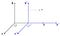Different frames of reference, including different positions and motions, would see different laws of physics (and would disagree on reality) if a theory is not relativistically invariant. The fact that we have a symmetry under ‘boosts,’ or velocity transformations, tells us we have a conserved quantity: linear momentum. The fact that a theory is invariant under any sort of coordinate or velocity transformation is known as Lorentz invariance, and any Lorentz invariant symmetry conserves CPT symmetry. However, C, P, and T (as well as the combinations CP, CT, and PT) may all be violated individually. (WIKIMEDIA COMMONS USER KREA)

In Einstein’s relativity, an observer who’s moving with respect to another observer will appear to have their lengths compressed and will appear to have their clocks run slow. These phenomena, known as length contraction and time dilation, were known even before Einstein, and have been experimentally verified under a wide variety of conditions to enormous precision. All observers agree: the laws of physics are the same for everyone, regardless of your position, velocity, or when in the Universe’s history you make your measurements.

But if there’s a minimum length scale to the Universe, that principle goes out the window, and leads to a paradox of two things that each must be true, but cannot be true together.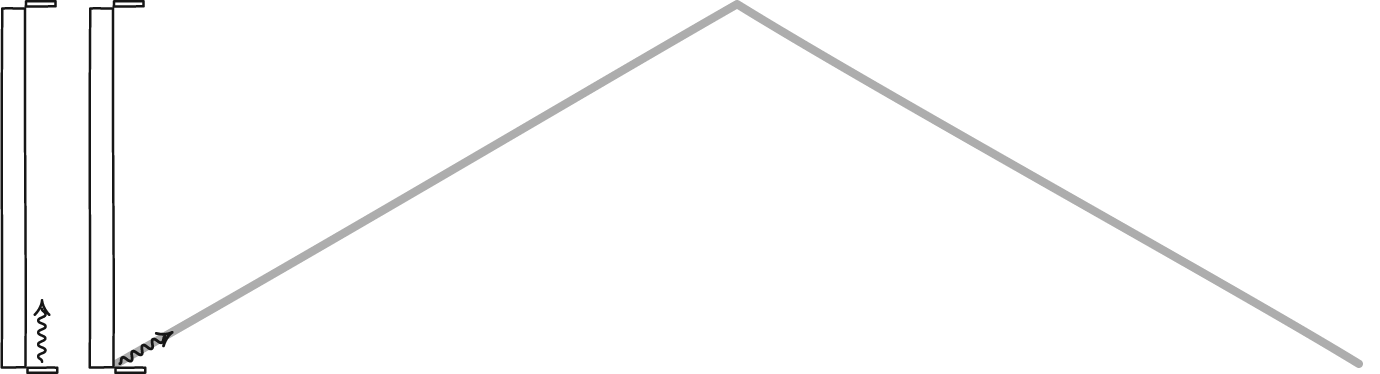A “light clock” will appear to run different for observers moving at different relative speeds, but this is due to the constancy of the speed of light. Einstein’s law of special relativity governs how these time and distance transformations take place between different observers. If there’s a fundamental length scale in one reference frame, an observer in a different reference frame will measure that fundamental scale to have a different, contracted length. (JOHN D. NORTON, VIA HTTP://WWW.PITT.EDU/~JDNORTON/TEACHING/HPS_0410/CHAPTERS/SPECIAL_RELATIVITY_CLOCKS_RODS/)

Imagine there’s a minimum length scale for someone at rest. Now, someone else comes along and starts moving close to the speed of light. According to relativity, that length that they’re looking at has to contract: it must be a smaller length than it is for someone at rest.

But if there’s a fundamental, minimum length scale, every observer should see that same minimum length. The laws of physics need to be the same for all observers, and that means everyone, regardless of how fast they’re moving.

That’s an enormous problem, because if there truly is a fundamental length scale, then different observers who move at different speeds relative to one another will observe that length scale to be different from one another. And if the fundamental length governing the Universe isn’t the same for everyone, than neither are the laws of physics.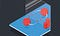We can imagine that there’s a mirror Universe to ours where the same rules apply. If the big red particle pictured above is a particle with an orientation with its momentum in one direction, and it decays (white indicators) through either the strong, electromagnetic, or weak interactions, producing ‘daughter’ particles when they do, that is the same as the mirror process of its antiparticle with its momentum reversed (i.e., moving backwards in time). If the mirror reflection under all three (C, P, and T) symmetries behaves the same as the particle in our Universe, then CPT symmetry is conserved. (CERN)

That’s a challenge for both theory and for experiment. Theoretically, if the laws of physics aren’t the same for everyone, then the principle of relativity is no longer valid. The CPT theorem, which states that every system in our Universe evolves identically to the same system where we’ve

• replaced all the particles with antiparticles (flipped the C-symmetry),

is now invalid. And the concept of Lorentz invariance, where all observers see the same laws of physics, has to go out the window as well. In General Relativity and the Standard Model, these symmetries are all perfect. If there’s a fundamental length scale to the Universe, one or both of them are, in some way, wrong.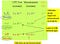The most stringest tests of CPT invariance have been performed on meson, lepton, and baryon-like particles. From these different channels, the CPT symmetry has been shown to be a good symmetry to precisions of better than 1-part-in-10-billion in all of them, with the meson channel reaching precisions of nearly 1 part in 10¹⁸. (GERALD GABRIELSE / GABRIELSE RESEARCH GROUP)

Experimentally, there are enormously tight constraints on violations of all of these. Particle physicists have probed the properties of matter and their antimatter counterparts under a huge range of experimental conditions, for stable, long-lived and short-lived particles. CPT has proven to be a good symmetry to better than 1 part in 10 billion for protons and antiprotons, better than 1 part in 500 billion for electrons and positrons, and better than 1 part in 500 quadrillion for kaons and anti-kaons.

Meanwhile, Lorentz invariance is observed to be a good symmetry from astrophysical constraints up to energies in excess of 100 billion GeV, or some 10 million times the energies reached at the Large Hadron Collider. A controversial but fascinating paper out just last month constrains Lorentz invariance violation to energies even beyond the Planck scale. If these symmetries are broken, the evidence has yet to show even a hint of appearing.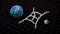Quantum gravity tries to combine Einstein’s General theory of Relativity with quantum mechanics. Quantum corrections to classical gravity are visualized as loop diagrams, as the one shown here in white. If you extend the Standard Model to include gravity, the symmetry that describes CPT (the Lorentz symmetry) may become only an approximate symmetry, allowing for violations. Thus far, however, no such experimental violations have been observed. (SLAC NATIONAL ACCELERATOR LAB)

In General Relativity, matter and energy determine the curvature of space and time, while spacetime curvature determines how matter and energy move through it. In both General Relativity and Quantum Field Theory, the laws of physics are the same everywhere and for everyone, regardless of their motion through the Universe. But if space has a fundamentally minimum length scale, then there is such a thing as a preferred frame of reference, and observers in motion relative to that frame of reference will obey different laws of physics from the preferred reference frame.

This doesn’t mean that gravity isn’t inherently quantum; space and time can be either continuous or discrete in a quantum Universe. But it means that if the Universe does have a fundamental length scale, that the CPT theorem, Lorentz invariance, and the principle of relativity must all be wrong. It could be so, but without the evidence to back it up, the idea of a fundamental length scale will remain speculative at best.

Starts With A Bang is now on Forbes, and republished on Medium on a 7-day delay. Ethan has authored two books, Beyond The Galaxy, and Treknology: The Science of Star Trek from Tricorders to Warp Drive.

Written by

Written by

## Ethan Siegel

#### The Universe is: Expanding, cooling, and dark. It starts with a bang! #Cosmology Science writer, astrophysicist, science communicator & NASA columnist.